# 如何解决过度拟合## 为何产生过度拟合

import numpy as np
import matplotlib.pyplot as plt

# Generate some data
x = np.linspace(0, 10, 100)
y = np.sin(x) + np.random.normal(scale=0.1, size=100)

# Fit a polynomial of degree 20
p = np.polyfit(x, y, 20)
y_pred = np.polyval(p, x)

# Plot the data and the fitted polynomial
plt.scatter(x, y)
plt.plot(x, y_pred, color='red')
plt.show()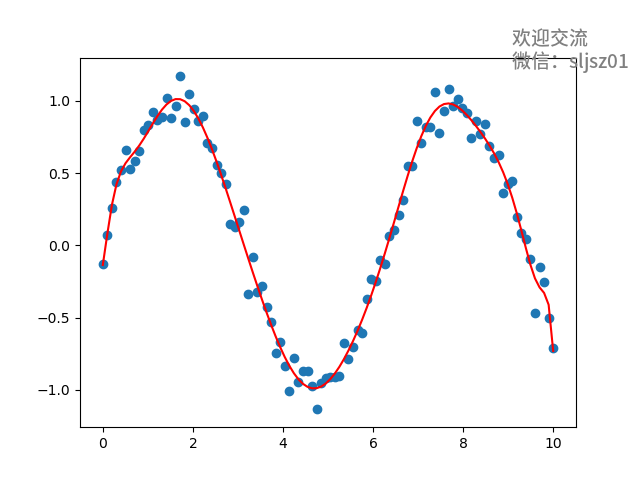## 如何解决过度拟合问题

from sklearn.model_selection import cross_val_score
from sklearn.linear_model import LinearRegression

# Generate some data
x = np.linspace(0, 10, 100)
y = np.sin(x) + np.random.normal(scale=0.1, size=100)

# Fit a polynomial of degree 20
p = np.polyfit(x, y, 20)
y_pred = np.polyval(p, x)

# Use linear regression with cross-validation to evaluate the model
lr = LinearRegression()
scores = cross_val_score(lr, x.reshape(-1, 1), y, cv=10)

# Print the mean score and standard deviation
print("Cross-validation scores:", scores)
print("Mean score:", np.mean(scores))
print("Standard deviation:", np.std(scores))

'''
output
Cross-validation scores: [-7.06062585e+00 -4.33284120e-04 -2.57612012e+01 -2.13349644e+00
-6.45893114e-01]
Mean score: -7.120329969404656
Standard deviation: 9.643292108330295
'''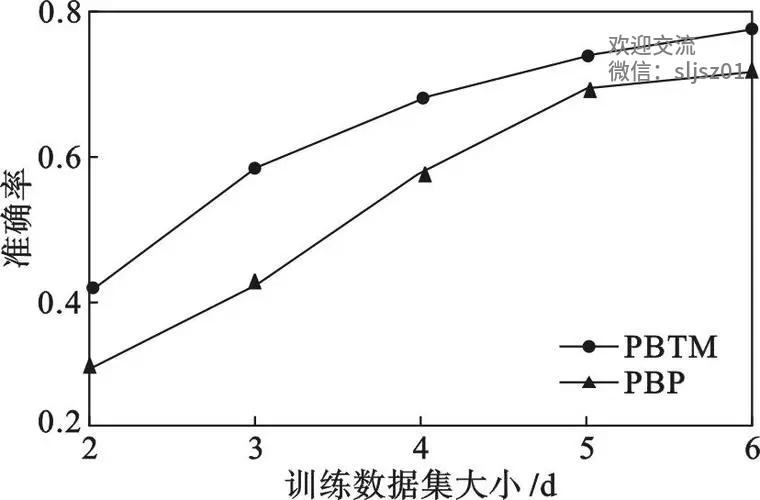# Import necessary libraries
import pandas as pd
from sklearn.model_selection import train_test_split
from sklearn.linear_model import LogisticRegression
from sklearn.feature_selection import SelectFromModel

# Split data into training and testing sets
X_train, X_test, y_train, y_test = train_test_split(data.drop('target', axis=1), data['target'], test_size=0.2, random_state=42)

# Create logistic regression model
lr = LogisticRegression()

# Use L1 regularization to select important features
selector = SelectFromModel(estimator=lr, threshold='1.25*median')
selector.fit(X_train, y_train)

# Transform training and testing sets to include only important features
X_train_selected = selector.transform(X_train)
X_test_selected = selector.transform(X_test)

# Fit logistic regression model on selected features
lr_selected = LogisticRegression()
lr_selected.fit(X_train_selected, y_train)

# Evaluate model performance on testing set
print('Accuracy on testing set:', lr_selected.score(X_test_selected, y_test))

## 对量化投资：实盘不如回测是过度拟合吗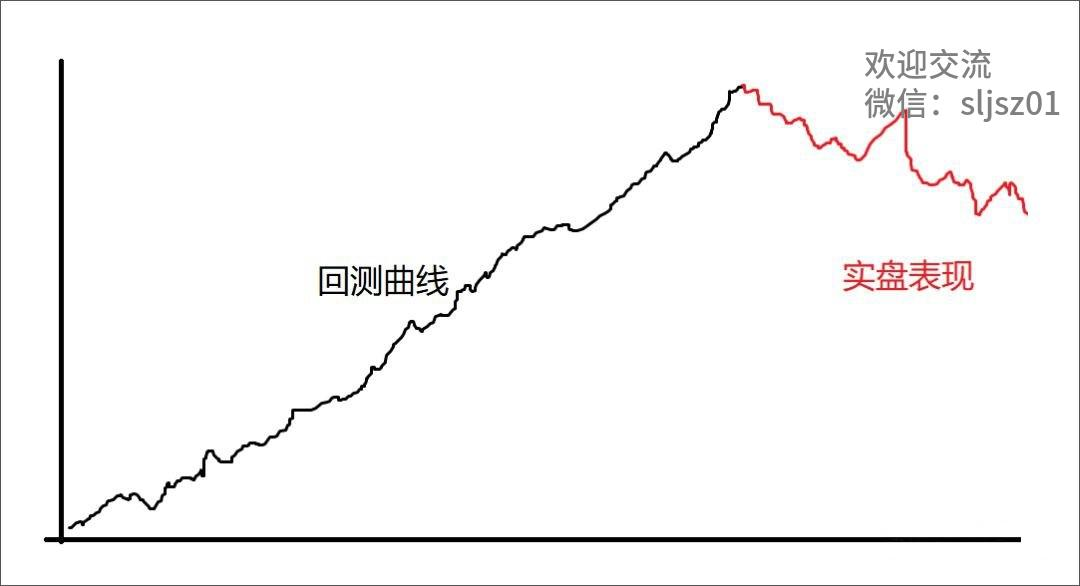1. 数据偏差：回测时使用的历史数据可能与实际市场环境存在一定的差异，例如我们在上文中提到的股指期货的例子，变化的市场结构会对策略的表现产生影响。

2. 滑点和交易成本：回测时通常假设买卖价格可以立即获得，但实际交易中存在滑点和交易成本，这些因素都可能会对策略的表现产生影响。

3. 策略实现：在实盘交易中，策略的实现可能会受到多种因素的影响，例如交易执行的速度、交易规模的限制等，这些因素也可能会对策略的表现产生影响。

4. 过度拟合：也是本文讨论的重点，在回测中，策略的过度拟合，使得策略在回测中表现良好，但在实际交易中表现不佳。

1. 尽可能使用更真实、更贴近目前市场状况的历史数据。

2. 在回测时保守的考虑交易成本和滑点等因素。

3. 在回测中尽可能遵循策略实盘交易的执行规则。

4. 采用交叉验证、扩充训练数据集、正则化特征选择、控制模型复杂度等有效方法，避免过度拟合。

## 写在最后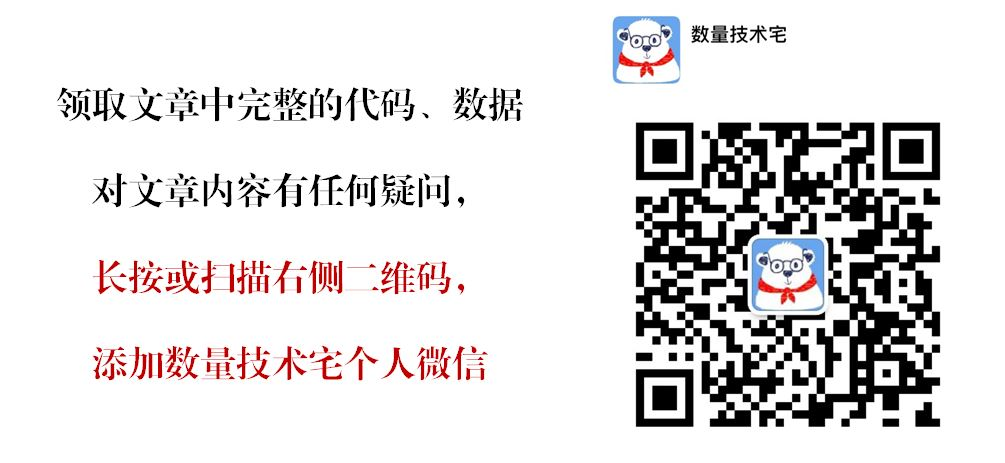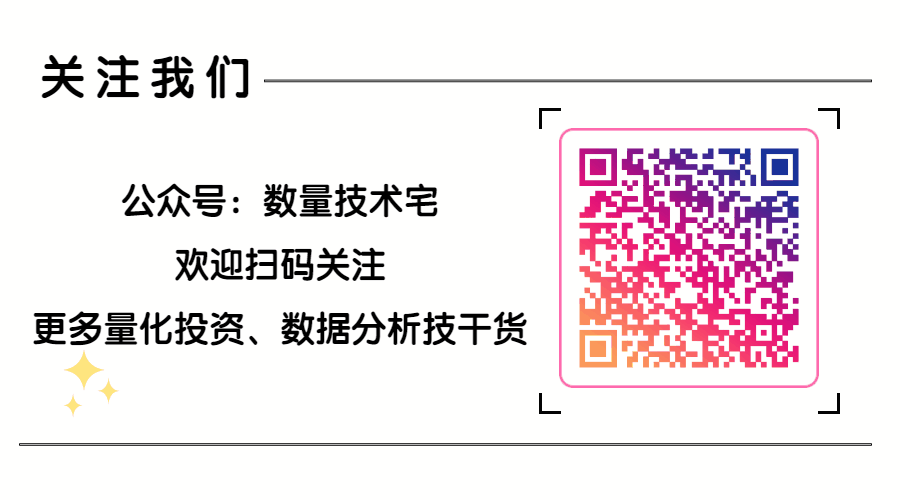posted @ 2023-05-14 09:59  数量技术宅  阅读(254)  评论(0编辑  收藏  举报